• Pytorch深度学习之Logistic回归 刘二大人Pytorch深度学习入门笔记及代码实现 文章目录Pytorch深度学习之Logistic回归前言一、为什么提出逻辑斯蒂回归？二、损失函数的选择三、代码实现 前言 本系列文章作为自己...
Pytorch深度学习之Logistic回归
刘二大人Pytorch深度学习入门笔记及代码实现

文章目录Pytorch深度学习之Logistic回归前言一、为什么提出逻辑斯蒂回归？二、损失函数的选择三、代码实现

前言
本系列文章作为自己深度学习入门的记录，该系列笔记参考刘二大人Pytorch深度学习实践课程实现，包括笔记与心得，一方面督促自己完成相关计划，另一方面有规律的记录加深自己对深度学习相关内容的理解。

提示：以下是本篇文章正文内容，下面案例可供参考
一、为什么提出逻辑斯蒂回归？

1、对于分类问题，模型经过训练，希望得到的结果是我们的输入到底属于哪一类，而对于线性回归，一般是在二维平面下估计输入x对应的输出y值。
正如下图所示：

x
y

1
2

2
4

3
6

4
？

线性回归希望得到一个表达式：$y = w * x + b$训练得到$w、b$的具体值。利用该式子可以对不同输入估算输出的值。分类问题不在追求y的具体数值为多少，更加希望得到一个关于输出$\hat y$的分布。分类问题，我们不能让模型训练的结果简单的输出$y = 0,1,2,3,...$原因很简单，分类问题不再属于线性问题，输入相似不代表输出就临近。以手写数字识别为例，7、9这两个数字输入很相似（形状相似）但是按照线性回归得到的表达式，各自对应的输出结果不会很相近，因为同一个模型下，输入8得到的结果和7、9更加接近。
这时我们可以知道分类问题的核心：根据不同的输入，得到对应不同输出（即分类类别）的概率分布，原来的求值变为求概率。$P(\hat y = i) = p_i$$\sum p_i = 1$
我们需要找到一个函数能够实现输出从具体值向概率分布的转变，有这样一类函数他们满足：

单调递增
有极限
是饱和函数
导数形状与正态分布相似
这一类函数称为Sigimod函数（激活函数）他可以把一个值从实数域映射到$y \in [0,1]$最常用的激活函数为Logistic函数：
$\Delta(x) =\cfrac{1}{1 + e^-x}$

函数图像为：这样在前馈网络中仅对输出进行变换就实现了从线性回归到分类问题的转变。
二、损失函数的选择
1.由于输出从原来的具体值变为概率分布，原有的MSE损失函数不在可取，因为模型得到的值限定在[0,1]之间，还是相减取平均的话得到的损失值很小，不利于模型参数的更新。
2.从构建损失函数的目的出发，我们希望利用这个式子反应模型训练值与真实值之间的差异，经过多次迭代训练，是的损失值趋近全局最小值。而每次训练损失函数值的变化决定的梯度下降的方向。
3.经过以上分析后我们选择交叉熵损失函数。
criterion = torch.nn.BCELoss(size_average=False)

三、代码实现
4.对于一个数据集：

x
y

1
0

2
0

3
1

4
？

利用深度学习框架实现二分类问题，代码如下：
import torch
import torch.nn.functional as F
x_data = torch.Tensor([[1.0], [2.0], [3.0]])
y_data = torch.Tensor([, , ])

class LinearModel(torch.nn.Module):
def __init__(self):
super(LinearModel, self).__init__()
self.linear = torch.nn.Linear(1, 1)

def forward(self, x):
y_pred = F.sigmoid(self.linear(x))
return y_pred
model = LinearModel()
#这个过程是构建计算图
criterion = torch.nn.BCELoss(size_average=False)
#对优化器进行类的实例化，不会构建计算图
optimizer = torch.optim.SGD(model.parameters(), lr= 0.01)
#进行训练
for ecoph in range(1000):
#进行前馈 model是实例化的模型类，是callable的对他进行传参数，直接可以调用进行计算
y_pred = model(x_data)
#计算实际损失
loss = criterion(y_pred, y_data)
print(ecoph ,loss.item())
#一定要进行梯度清零，计算一次损失后就会相应计算梯度，如果不清零就会将梯度累加，导致参数更新出现问题
#进行反馈更新参数
loss.backward()
optimizer.step()
print("w= ", model.linear.weight.item())
print("b= ", model.linear.bias.item())

x_test = torch.Tensor([4.0])
y_test = model(x_test)
print("y_pred =", y_test.item())

代码得到结果：展开全文机器学习
• ## Pytorch深度学习入门

千次阅读 多人点赞 2018-12-08 21:03:37
title: Pytorch深度学习入门 mathjax: false date: 2018-12-06 12:34:12 categories: - 深度学习 tags: - 深度学习 - Pytorch 在本教程中，将使用PyTorch框架介绍深度学习，并通过一个案例进行实验，通过本教程，你...
在本教程中，将使用Pytorch框架介绍深度学习，并通过一个简单案例进行实验，通过本教程，你将可以轻松地使用Pytorch框架构建深度学习模型。（我也刚刚接触Pytorch）
Pytorch 简介
Pytorch 是一个基于 Torch 的 Python 机器学习包，而 Torch 则是一个基于编程语言 Lua 的开源机器学习包。Pytorch 有两个主要的特点：

利用强大的 GPU 加速进行张量计算（如 NumPy）
用于构建和训练神经网络的自动微分机制

相比其它深度学习库，Pytorch 具有以下两点优势：

与 TensorFlow 等其它在运行模型之前必须先定义整个计算图的库不同，Pytorch 允许动态定义图。
Pytorch 也非常适合深度学习研究，提供了最大的灵活性和运行速度。

Tensors
Pytorch 张量与 NumPy 数组非常相似，而且它们可以在 GPU 上运行。这一点很重要，因为它有助于加速数值计算，从而可以将神经网络的速度提高 50 倍甚至更多。为了使用 Pytorch，你需要先访问其官网并安装 Pytorch。如果你正在使用 Conda，你可以通过运行以下简单命令来安装Pytorch：
conda install PyTorch torchvision -c PyTorch

为了定义 Pytorch 张量，首先需要导入 torch 包。PyTorch 允许你定义两种类型的张量，即 CPU 和 GPU 张量。在本教程中，假设你运行的是使用 CPU 进行深度学习运算的机器，但我也会向你展示如何在 GPU 中定义张量：
import torch

Pytorch 的默认张量类型是一个浮点型张量，定义为torch.FloatTensor。例如，你可以根据 Python 的 list 数据结构创建张量：
torch.FloatTensor([[20, 30, 40], [90, 60, 70]])

如果你使用的是支持 GPU 的机器，你可以通过以下方法定义张量：
torch.cuda.FloatTensor([[20, 30, 40], [90, 60, 70]])

你也可以使用 Pytorch 张量执行加法和减法等数学运算：
x = torch.FloatTensor()
y = torch.FloatTensor()
x + y

你还可以定义矩阵并执行矩阵运算。我们来看看如何定义一个矩阵然后将其转置：
matrix = torch.randn(4, 5)
matrix
matrix.t()

Pytorch 使用了一种叫做自动微分的技术，它可以对函数的导数进行数值估计。自动微分在神经网络中计算反向传递（backward pass）。在训练过程中，神经网络的权重被随机初始化为接近零但不是零的数。反向传递是指从右到左调整权重的过程，而正向传递则是从左到右调整权重的过程。
torch.autograd是 Pytorch 中支持自动微分的库。这个包的核心类是torch.Tensor。如果你想要跟踪这个类的所有操作，请将.requires_grad设置为 True。如果要计算所有的梯度，请调用.backward()。这个张量的梯度将在.grad属性中积累。
如果你想要从计算历史中分离出一个张量，请调用.detach()函数。这也可以防止将来对张量的计算被跟踪。另一种防止历史跟踪的方法是用torch.no_grad()方法封装代码。
你可以将张量Tensor和函数Function类相连接，构建一个编码了完整计算历史的无环图。张量的.grad_fn属性会引用创建了这个张量的Function。如果你要计算导数，可以调用张量的.backward()。如果该张量包含一个元素，你不需要为backward()函数指定任何参数。如果张量包含多个元素，你需要指定一个规模（shape）相匹配的张量的梯度。
例如，你可以创建两个张量，将其中一个张量的requires_grad设定为 True，将另一个的设定为 False。接着你可以用这两个张量来执行加法和求和运算。然后你可以计算其中一个张量的梯度。
a = torch.tensor([3.0, 2.0], requires_grad=True)
b = torch.tensor([4.0, 7.0])
ab_sum = a + b
ab_sum
ab_res = (ab_sum*8).sum()
ab_res.backward()
ab_res

在b上调用.grad的返回值为空，因为你没有将它的requires_grad设置为 True。
nn 模块
这是在 Pytorch 中构建神经网络的模块。nn模块依赖于autograd来定义模型并对其进行微分处理。首先，定义训练一个神经网络的过程：

用一些可学习的参数（即权重）定义神经网络
在输入的数据集上进行迭代
通过网络处理输入
将预测结果和实际值进行比较，并测量误差
将梯度传播回网络的参数中
使用简单的更新规则更新网络的权重：weight = weight - learning_rate * gradient

现在，你可以使用nn模块创建一个双层的神经网络：
N, D_in, H, D_out = 64, 1000, 100, 10
x = torch.randn(N, D_in)
y = torch.randn(N, D_out)
model = torch.nn.Sequential(
torch.nn.Linear(D_in, H),
torch.nn.ReLU(),
torch.nn.Linear(H, D_out),
)
loss_fn = torch.nn.MSELoss()
learning_rate = 1e-4

在这里我们将解释一下上面用到的参数：

N 是批处理大小。批处理大小是观测数据的数量，观测之后权重将被更新。

D_in: 是输入的维度

H: 是隐藏层的维度

D_out: 是输出层的维度

torch.randn: 定义了指定维度的矩阵

torch.nn.Sequential :初始化了神经网络层的线性堆栈

torch.nn.Linear: 对输入数据应用了线性变换

torch.nn.ReLU:  在元素层级上应用了ReLU激活函数

torch.nn.MSELoss: 创建了一个标准来度量输入 x 和目标 y 中 n 个元素的均方误差

optim 模块
该优化器接受的第一个参数是张量，这些张量需要更新。在正向传递中，你要通过向模型传递 x 来计算出预测的 y。然后，计算并显示出损失。在运行反向传递之前，你要将使用优化器更新的所有变量的梯度设置为零。这样做的原因是，默认情况下，在调用.backward()方法时，梯度不会被重写。然后，你需要在优化器上调用step函数，该步骤会更新其参数。具体的实现代码如下所示：
 optimizer = torch.optim.Adam(model.parameters(), lr=learning_rate)
for t in range(500):
y_pred = model(x)
loss = loss_fn(y_pred, y)
print(t, loss.item())
loss.backward()
optimizer.step()

自定义的 nn 模块
有时你需要构建自己的自定义模块。这种情况下，你需要创建nn.Module的子类，然后定义一个接收输入张量并产生输出张量的 forward。使用nn.Module实现双层网络的方法如下图所示。这个模型与上面的模型非常相似，但不同之处在于你要使用torch.nn.Module创建神经网络。另一个区别是这个模型会使用 stochastic gradient descent optimizer 而不是 Adam。你可以使用下面的代码实现一个自定义的 nn 模块：
import torch
class TwoLayerNet(torch.nn.Module):
def __init__(self, D_in, H, D_out):
super(TwoLayerNet, self).__init__()
self.linear1 = torch.nn.Linear(D_in, H)
self.linear2 = torch.nn.Linear(H, D_out)
def forward(self, x):
h_relu = self.linear1(x).clamp(min=0)
y_pred = self.linear2(h_relu)
return y_pred
N, D_in, H, D_out = 64, 1000, 100, 10
x = torch.randn(N, D_in)
y = torch.randn(N, D_out)
model = TwoLayerNet(D_in, H, D_out)
criterion = torch.nn.MSELoss()
optimizer = torch.optim.SGD(model.parameters(), lr=1e-4)
for t in range(500):
y_pred = model(x)
loss = criterion(y_pred, y)
print(t, loss.item())
loss.backward()
optimizer.step()

案例
上面介绍完一些Pytorch的基础之后，接下来，我们将通过一个完整地案例进一步加深对Pytorch的认识。
我们将按照下列目录进行实验:
--- pytorchFashion
| |--- __init__.py
| |--- callback
| |--- config
| |--- dataset
| |--- output
| |--- test
| |--- train
| |--- io
| |--- model
| | |--- __init__.py
| | |--- cnn
| | | |--- __init__.py
| | | |--- alexnet.py
| |--- preprocessing
| |--- utils

简单地对每个目录进行说明，其中:

callback: 我们自定义的callback
config: 整个实验的配置文件目录
dataset: 数据集目录
output:实验输出目录
test: 预测器目录
train:训练器目录
io: 数据交互目录
model: 模型目录
preprocessing: 数据预处理目录
utils: 常用工具目录

我们主要以常见的FashionMNIST数据集进行实验。
FashionMNIST
FashionMNIST 是一个替代 MNIST 手写数字集 的图像数据集。 它是由 Zalando（一家德国的时尚科技公司）旗下的研究部门提供。其涵盖了来自 10 种类别的共 7 万个不同商品的正面图片。
FashionMNIST 的大小、格式和训练集/测试集划分与原始的 MNIST 完全一致。60000/10000 的训练测试数据划分，28x28 的灰度图片。你可以直接用它来测试你的机器学习和深度学习算法性能，且不需要改动任何的代码。说白了就是手写数字没有衣服鞋子之类的更复杂。
数据案例如下所示：备注: 该数据集可以从地址进行下载。
实际上，Pytorch模块中已经包含了下载以及处理FashionMNIST数据的脚本，我们只需运行:
    transform = transforms.Compose([transforms.ToTensor(),
transforms.Normalize((0.5, 0.5, 0.5), (0.5, 0.5, 0.5))])
train_dataset = datasets.FashionMNIST(root='./dataset/fashion',
train=True,
transform=transform)
test_dataset = datasets.FashionMNIST(root='./dataset/fashion',
train=False,
transform=transform)
batch_size=batch_size,
shuffle=True)
batch_size=batch_size,
shuffle=False)

各个模块的代码可以从github上获取，这里将不详细描述。
我们主要实现run.py脚本文件，详细代码可看源文件。在pytorchFashion同目录下新建一个run.py文件，并写入以下代码：
#encoding:utf-8
import argparse
import torch
import numpy as np
import torchvision.transforms as transforms
import torchvision.datasets as datasets
from pytorchFashion.train.trainer import Trainer
from pytorchFashion.utils.logginger import init_logger
from pytorchFashion.config import alexnet_config as config
from pytorchFashion.train.losses import CrossEntropy
from pytorchFashion.train.metrics import Accuracy
from pytorchFashion.callback.lrscheduler import StepLr
from pytorchFashion.model.cnn.alexnet import AlexNet
from pytorchFashion.callback.earlystopping import EarlyStopping
from pytorchFashion.callback.modelcheckpoint import ModelCheckpoint
from pytorchFashion.callback.trainingmonitor import TrainingMonitor
from pytorchFashion.callback.writetensorboard import WriterTensorboardX

首先，我们加载所需模块，可以看到大部分都是我们自定义的模块。接下里，我们定义一个执行的主函数main()，如下:
def main():
# 路径变量
checkpoint_dir = config.CHECKPOINT_PATH # checkpoint路径
fig_path = config.FIG_PATH
json_path = config.JSON_PATH
# 初始化日志
logger = init_logger(log_name=config.ARCH,
log_path=config.LOG_PATH)
if args['seed'] is not None:
logger.info("seed is %d"%args['seed'])
np.random.seed(args['seed'])
torch.manual_seed(args['seed'])

其中 :

checkpoint_dir：模型保存路径
fig_path：训练结果可视化保存路径
json_path：训练指标变化保存路径

另外，我们还初始化一个日志记录器logger，记录整个实验过程。以及一个固定的随机种子变量seed（保证结果可复现）。
接着，加载数据，即:
    # 加载数据集
logger.info('starting load train data from disk')
transform = transforms.Compose([transforms.ToTensor(),
transforms.Normalize((0.5, 0.5, 0.5), (0.5, 0.5, 0.5))])
train_dataset = datasets.FashionMNIST(root='./dataset/fashion',
train=True,
transform=transform)
test_dataset = datasets.FashionMNIST(root='./dataset/fashion',
train=False,
transform=transform)
batch_size=config.BATCH_SIZE,
shuffle=True)
batch_size=config.BATCH_SIZE,
shuffle=False)

其中，transform负责整个数据转换过程，需要注意的是transforms.ToTensor()将变量转化为张量时，默对每张图片像素除以256操作，所以，你会看到我们在进行归一化时，传入的各个通道均值位于0到1之间。
定义好数据之后，接下来，初始化模型和优化器，即：
    # 初始化模型和优化器
logger.info("initializing model")
model = SimpleNet(num_classes = config.NUM_CLASSES)
lr = config.LEARNING_RATE,
weight_decay=config.WEIGHT_DECAY,
)

下面，初始化callback模块:
 # 写入TensorBoard
logger.info("initializing callbacks")
write_summary = WriterTensorboardX(writer_dir=config.WRITER_PATH,
logger = logger,
enable=True)
# 模型保存
model_checkpoint = ModelCheckpoint(checkpoint_dir=checkpoint_dir,
mode= config.MODE,
monitor=config.MONITOR,
save_best_only= config.SAVE_BEST_ONLY,
arch = config.ARCH,
logger = logger)
# eraly_stopping功能
early_stop = EarlyStopping(mode = config.MODE,
patience = config.PATIENCE,
monitor = config.MONITOR)
# 监控训练过程
train_monitor = TrainingMonitor(fig_path = fig_path,
json_path = json_path,
arch = config.ARCH)
lr_scheduler = StepLr(optimizer=optimizer,lr = config.LEARNING_RATE)

其中:

write_summary: 主要是负责将数据写入文件中，以便于使用tensorboard工具进行可视化。
model_checkpoint： 保存模型，这里默认是保存最佳模型，当然也可以指定epoch频率保存模型。
early_stop：当模型在训练过程中，如果模型持续一段时间不再学习，那么使用该功能可以自动停止训练模型。
train_monitor：模型训练过程中的监控器，主要记录各个指标变化情况。
lr_scheduler：学习率变化模式，我们知道一般刚开始训练模型时，应设置一个大的学习率，当模型不断接近最小值时，应设置一个小的学习率。

初始化模型训练器:
    logger.info('training model....')
trainer = Trainer(model = model,
train_data = trainIter,
val_data = valIter,
optimizer = optimizer,
criterion=CrossEntropy(),
metric = Accuracy(topK=config.TOPK),
logger = logger,
config = config,
model_checkpoint = model_checkpoint,
training_monitor = train_monitor,
early_stopping = early_stop,
writer= write_summary,
train_from_scratch=config.RESUME,
lr_scheduler=lr_scheduler
)

开始训练模型:
    # 查看模型结构
trainer.summary()
# 拟合模型
trainer.train()
if __name__ == '__main__':
ap = argparse.ArgumentParser(description='PyTorch model training')
help = 'seed for initializing training.')
args = vars(ap.parse_args())
main()


以上，我们完成了整个训练模型脚本，接下来运行下列命令进行模型训练:
python run.py

在训练结束之后，在output目录，你可以看到两个文件，分别记录了loss和accuracy的变化情况，即: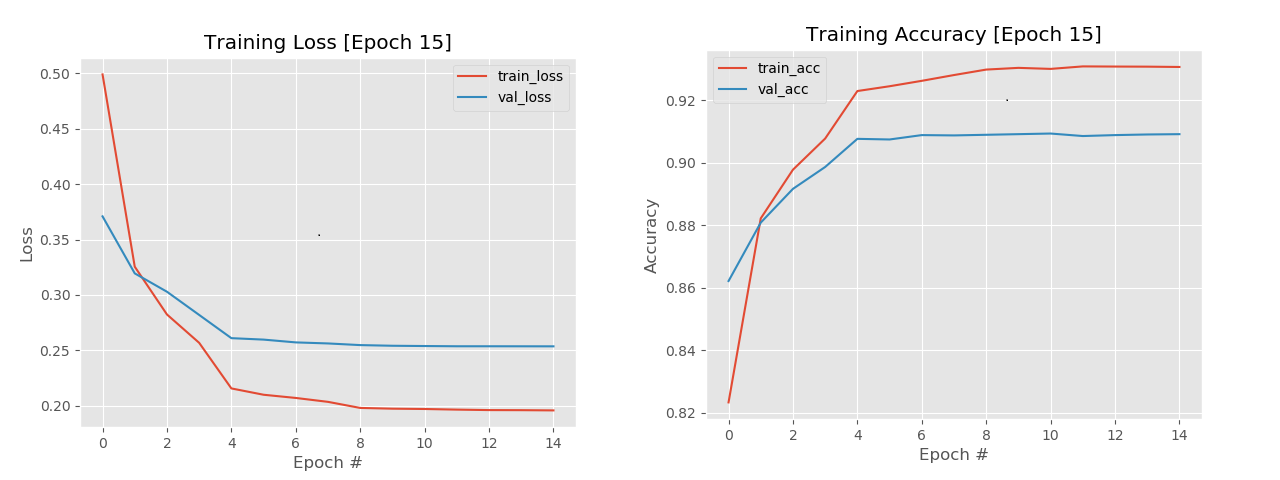备注：完整代码可从:github下载


展开全文• PyTorch学习笔记6—PyTorch深度学习入门（四）—入门实例
PyTorch学习笔记6—PyTorch深度学习入门（四）—入门实例4. 实例：训练cifar10图像分类器4.1 前言：关于数据4.1.1 Dataset4.1.2 Datalorder4.1.3 torchvision 包4.1.3.1 transforms4.1.3.2 datasets4.1.3.3 models4.2 实例代码

本篇是pytorch学习笔记系列第六篇，这一系列博客应该大多会来自于开源教程书：pytorch中文手册（pytorch handbook），其github网址为：https://github.com/zergtant/pytorch-handbook  本篇博客内容来自于这一手册以及pytorch官方的教程 ，感兴趣的观众门可以去自行下载

4. 实例：训练cifar10图像分类器
4.1 前言：关于数据
一般情况下处理图像，文本，音频和视频数据时，可以使用标准的Python包来加载数据到一个numpy数组中. 然后把这个数组转换成 torch.*Tensor。其中：

图像可以使用 Pillow, OpenCV
音频可以使用 scipy , librosa
文本可以使用原始Python和Cython来加载,或者使用 NLTK或 SpaCy 处理

4.1.1 Dataset
Dataset是一个抽象类, 为了能够方便的读取，需要将要使用的数据包装为Dataset类。 自定义的Dataset需要继承它并且实现两个成员方法：

getitem() ：该方法定义每次怎么获取数据
len() ：该方法返回数据集的总长度

下面我们使用kaggle上的一个竞赛bluebook for bulldozers自定义一个数据集，为了方便介绍，我们使用里面的数据字典来做说明（因为条数少）
下面我们使用kaggle上的一个竞赛bluebook for bulldozers自定义一个数据集，为了方便介绍，我们使用里面的数据字典来做说明（因为条数少）
from torch.utils.data import Dataset
import pandas as pd
#定义一个数据集
class BulldozerDataset(Dataset):
""" 数据集演示 """
def __init__(self, csv_file):
"""实现初始化方法，在初始化的时候将数据读载入"""
def __len__(self):
'''
返回df的长度
'''
return len(self.df)
def __getitem__(self, idx):
'''
根据IDX返回一列数据
'''
return self.df.iloc[idx].SalePrice

至此，我们的数据集已经定义完成了，我们可以实例话一个对象访问他
ds_demo= BulldozerDataset('median_benchmark.csv')
len(ds_demo)#实现了__len__ 方法所以可以直接使用len获取数据总数
ds_demo#用索引可以直接访问对应的数据

得到以下输出：
11573
24000.0

4.1.2 Datalorder
dl = torch.utils.data.DataLoader(ds_demo, batch_size=10, shuffle=True, num_workers=0)DataLoader #返回的是一个迭代器，我们可以使用迭代器分次获取数据
idata=iter(dl)
print(next(idata))
for i, data in enumerate(dl):
print(i,data)
#这里只循环一遍
break

输出结果：
tensor([24000., 24000., 24000., 24000., 24000., 24000., 24000., 24000., 24000.,
24000.], dtype=torch.float64)

0 tensor([24000., 24000., 24000., 24000., 24000., 24000., 24000., 24000., 24000.,
24000.], dtype=torch.float64)

可以看到：我们已经可以通过dataset定义数据集，并使用Datalorder载入和遍历数据集，
4.1.3 torchvision 包
4.1.3.1 transforms
transforms 模块提供了一般的图像转换操作类，用作数据的处理和增广，如下例定义了一个图像转换器：
from torchvision import transforms as transforms
transform = transforms.Compose([
transforms.RandomHorizontalFlip(),  #图像一半的概率翻转，一半的概率不翻转
transforms.RandomRotation((-45,45)), #随机旋转
transforms.ToTensor(),
transforms.Normalize((0.4914, 0.4822, 0.4465), (0.229, 0.224, 0.225)), #R,G,B每层的归一化用到的均值和方差
])

肯定有人会问：(0.485, 0.456, 0.406), (0.2023, 0.1994, 0.2010) 这几个数字是什么意思？
官方的这个帖子有详细的说明: https://discuss.pytorch.org/t/normalization-in-the-mnist-example/457/21 这些都是根据ImageNet训练的归一化参数，可以直接使用，pytorch认为这个是固定值就可以
4.1.3.2 datasets
torchvision.datasets包包含很多数据集，可以理解为PyTorch团队自定义的dataset，这些dataset帮我们提前处理好了很多的图片数据集，我们拿来就可以直接使用：

MNIST
COCO
Captions
Detection
LSUN
ImageFolder
Imagenet-12
CIFAR
STL10
SVHN
PhotoTour

如下例所示导入了MNIST数据集
import torchvision.datasets as datasets
trainset = datasets.MNIST(root='./data', # 表示 MNIST 数据的加载的目录
train=True,  # 表示是否加载数据库的训练集，false的时候加载测试集
transform=None) # 表示是否需要对数据进行预处理，none为不进行预处理

4.1.3.3 models
torchvision不仅提供了常用图片数据集，还提供了训练好的模型，可以加载之后，直接使用，或者在进行迁移学习 torchvision.models模块的 子模块中包含以下模型结构。

AlexNet
VGG
ResNet
SqueezeNet
DenseNet

我们直接可以使用训练好的模型，当然这个与datasets相同，都是需要从服务器下载的
如下例所示将导入resnet18这个网络模型
import torchvision.models as models
resnet18 = models.resnet18(pretrained=True)

所以说，torchvision包不仅提供了巨大的便利,也避免了代码的重复.
4.2 实例代码
在这个实例中,我们使用CIFAR10数据集,它有如下10个类别：‘airplane’, ‘automobile’, ‘bird’, ‘cat’, ‘deer’, ‘dog’, ‘frog’, ‘horse’, ‘ship’, ‘truck’. CIFAR-10的图像都是 3x32x32大小的，即3颜色通道，32*32像素.
训练一个图像分类器，将依次按照下列顺序进行:

使用torchvision加载和归一化CIFAR10训练集和测试集
定义一个卷积神经网络
定义损失函数
在训练集上训练网络
在测试集上测试网络

import torch
import torchvision
import torchvision.transforms as transforms

import matplotlib.pyplot as plt
import numpy as np

import torch.nn as nn

import torch.optim as optim

import time
start =time.process_time()

# 展示图像的函数
def imshow(img):
img = img / 2 + 0.5     # unnormalize
npimg = img.cpu().numpy()
plt.imshow(np.transpose(npimg, (1, 2, 0)))
plt.show()

'''1.读取数据集'''
#torchvision的输出是[0,1]的PILImage图像,我们把它转换为归一化范围为[-1, 1]的张量
transform = transforms.Compose(
[transforms.ToTensor(),
transforms.Normalize((0.5, 0.5, 0.5), (0.5, 0.5, 0.5))])
#print(transform)
#读取数据集
classes = ('plane', 'car', 'bird', 'cat','deer', 'dog', 'frog', 'horse', 'ship', 'truck')

'''测试数据集是否可用'''
# 获取随机数据
images, labels = dataiter.next()

# 展示图像
imshow(torchvision.utils.make_grid(images))
# 显示图像标签
print(' '.join('%5s' % classes[labels[j]] for j in range(4)))

# 确认我们的电脑支持 CUDA ,然后显示CUDA信息:
device = torch.device("cuda:0" if torch.cuda.is_available() else "cpu")
print(device)

'''2.定义卷积神经网络'''
class Net(nn.Module):
def __init__(self):
super(Net, self).__init__()
self.conv1 = nn.Conv2d(3, 6, 5)
self.pool = nn.MaxPool2d(2, 2)
self.conv2 = nn.Conv2d(6, 16, 5)
self.fc1 = nn.Linear(16 * 5 * 5, 120)
self.fc2 = nn.Linear(120, 84)
self.fc3 = nn.Linear(84, 10)

def forward(self, x):
x = self.pool(F.relu(self.conv1(x)))
x = self.pool(F.relu(self.conv2(x)))
x = x.view(-1, 16 * 5 * 5)
x = F.relu(self.fc1(x))
x = F.relu(self.fc2(x))
x = self.fc3(x)
return x

#获得Net变量
net = Net()
#将递归遍历所有模块并将模块的参数和缓冲区 转换成CUDA张量的：
net.to(device)

'''3.定义损失函数和优化器
使用交叉熵作为损失函数,使用带动量的随机梯度下降'''
criterion = nn.CrossEntropyLoss()
optimizer = optim.SGD(net.parameters(), lr=0.001, momentum=0.9)

'''4.训练网路'''
for epoch in range(2):  # 多批次循环

running_loss = 0.0
for i, data in enumerate(trainloader, 0):
# 获取输入
inputs, labels = data
# inputs 和targets 也要转换
inputs, labels = inputs.to(device), labels.to(device)
# 梯度置0

# 正向传播，反向传播，优化
outputs = net(inputs)
loss = criterion(outputs, labels)
loss.backward()
optimizer.step()

# 打印状态信息
running_loss += loss.item()
if i % 2000 == 1999:    # 每2000批次打印一次
print('[%d, %5d] loss: %.3f' %
(epoch + 1, i + 1, running_loss / 2000))
running_loss = 0.0

print('Finished Training')

'''5.测试集测试网络'''
images, labels = dataiter.next()
images, labels = images.to(device), labels.to(device)

#5.1 用几张图片来测试
# 显示图片和标签
imshow(torchvision.utils.make_grid(images))
print('GroundTruth: ', ' '.join('%5s' % classes[labels[j]] for j in range(4)))
#神经网络的预测结果
outputs = net(images)
_, predicted = torch.max(outputs, 1)
print('Predicted: ', ' '.join('%5s' % classes[predicted[j]] for j in range(4)))

#5.2 查看网络在测试集上的准确率
correct = 0
total = 0
images, labels = data
# inputs 和targets 也要转换
images, labels = images.to(device), labels.to(device)
outputs = net(images)
_, predicted = torch.max(outputs.data, 1)
total += labels.size(0)
correct += (predicted == labels).sum().item()

print('Accuracy of the network on the 10000 test images: %d %%' % (100 * correct / total))

#5.3 查看网络在各个类别测试的准确率
class_correct = list(0. for i in range(10))
class_total = list(0. for i in range(10))
images, labels = data
# inputs 和targets 也要转换
images, labels = images.to(device), labels.to(device)
outputs = net(images)
_, predicted = torch.max(outputs, 1)
c = (predicted == labels).squeeze()
for i in range(4):
label = labels[i]
class_correct[label] += c[i].item()
class_total[label] += 1

for i in range(10):
print('Accuracy of %5s : %2d %%' % (classes[i], 100 * class_correct[i] / class_total[i]))

#输出程序运行时间
end = time.process_time()
print('Running time: %s Seconds'%(end-start))

上例的代码是在GPU中运行的，若要改成CPU运行，需将其中的.to方法去掉
如果有多个GPU，需要数据并行，则在定义网络对象后，可以使用DataParallel方法让模型并行运行在多个GPU上。
net= nn.DataParallel(net)

至此，我们已经使用pytorch完成了一个深度学习的编程全过程，进行了初步的入门：使用torchvision加载和归一化CIFAR10训练集和测试集、定义一个卷积神经网络、定义损失函数、在训练集上训练网络、在测试集上测试网络。
更加深入的的案例，比如其他神经网络、其他损失函数和优化器，将在之后的学习笔记中记录。
本篇完…
本系列已更新的学习笔记：
PyTorch学习笔记1—PyTorch简介
PyTorch学习笔记2—win10下pytorch-gpu安装以及CUDA安装记录
PyTorch学习笔记3—PyTorch深度学习入门（一）—基本方法
PyTorch学习笔记4—PyTorch深度学习入门（二）—自动求导
PyTorch学习笔记5—PyTorch深度学习入门（三）—神经网络
PyTorch学习笔记6—PyTorch深度学习入门（四）—入门实例


展开全文神经网络 python 人工智能
• 第6章　序列数据和文本的深度学习 在上一章中，我们讨论了如何利用卷积神经网络处理带有空间信息的数据，以及如何构建图像分类器。本章将讨论以下主题： 用于构建深度学习模型的不同文本数据表示法： 理解递归神经...
• 刘老师B站《PyTorch深度学习时实践课程》的P6-P9的相关笔记和代码
PyTorch深度学习实践
逻辑斯蒂回归及实现

背景与概念

基于分类问题中属性是类别性的，所以不能采取基于序数的线性回归模型，而提出了新的分类模型——逻辑斯蒂回归模型，输出每个样本在各个预测值上的概率值。
为了将最终的输出值控制在概率值P∈[0,1]的合理范围中，需要在线性单元输出后再加上一个非线性映射，这里我们使用饱和函数sigmoid
因为模型发生了变化，损失值的计算方式也要发生相应的变化；因为线性回归模型只需要比较两个实数之间的差异，所以可以使用均方误差值衡量；但是逻辑回归得到的结果是在描述事件的概率分布，因此我们的误差函数也变成比较两个分布之间的差异，这里我们使用交叉熵计算公式。

代码实现

import numpy as np
import torch
import torch.nn.functional as F
import matplotlib.pyplot as plt

#二分类问题的输出值往往是0或1
x_data = torch.tensor([[1.0],[2.0],[3.0]])
y_data = torch.tensor([[0.0],[0.0],[1.0]])

#构建训练模型
class LogisticRegressionModel(torch.nn.Module):
def __init__(self):
super(LogisticRegressionModel,self).__init__()#这一块和线性回归的模型差距不大，因为sigmoid函数是无参数的，不需进行初始化
self.linear = torch.nn.Linear(1,1)

def forward(self,x):
y_pred = F.sigmoid(self.linear(x))#比线性回归模型就多了一步非线性映射
return y_pred

model = LogisticRegressionModel()

#构建损失函数的计算和优化器
criterion = torch.nn.BCELoss(size_average = False)#逻辑回归模型适用二分类交叉熵损失函数
op = torch.optim.SGD(model.parameters(),lr = 0.01)

epochs = []
costs = []
#训练过程，对每个优化器都进行尝试
for epoch in range(1000):
epochs.append(epoch)
y_pred = model(x_data)
loss = criterion(y_pred, y_data)
costs.append(loss.item())
print(epoch, loss.item())

loss.backward()
op.step()

# 对训练结果进行输出
print('w = ', model.linear.weight.item())
print('b = ', model.linear.bias.item())

# 进行模型测试
X = np.linspace(0,10,200)
x_test = torch.Tensor(X).view(200,1)
y_test = model(x_test)
Y = y_test.data.numpy()
plt.ylabel('Probability of Pass')
plt.xlabel('Hours')
plt.plot(X, Y)
plt.grid()
plt.show()

# 训练过程可视化
plt.ylabel('Cost')
plt.xlabel('Epoch')
plt.plot(epochs, costs)
plt.show()

多维特征的输入

输入特征的变化

所谓输入特征的维度变化，影响的只是线性单元中控制输入维度的那一个参数 ；
在之前讲的例子中，每一个线性单元所做的工作即对一个实数输入x，乘上一个权重之后再加上一个偏置项，（之后再进行非线性映射），在这种情况下——输入的数据维度是1，线性单元的权重维度也是1x1大小的；

在实际的问题中，一个数据项含有多种特征属性且都与该问题相关联，此时我们输入的不再是一个实数，而是一个向量，同样权重单元也不再是1x1的，而也应该是一个相应维度的向量；输入与权重的运算就转换成向量之间的点乘运算，数与数之间的四则运算也同理转换成向量/矩阵的四则运算。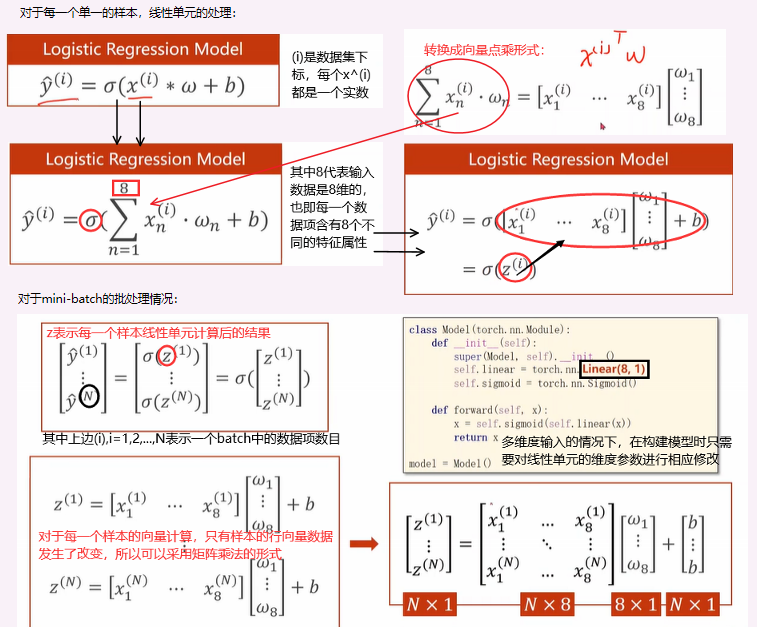神经网络的本质：找到合适的空间变换

①从前面的讨论中，我们不难发现，如果将一个M维的数据传入线性计算单元，得到N维的输出，那么实际上是在做一个矩阵线性变换——从M维空间映射到N维空间。

②神经网络的学习面向的大多是现实社会中的问题，大多是要找到不同维度空间的非线性映射，我们采用将一层线性映射分解成多层线性映射（这不会改变线性映射的性质）并在每一层映射后加入非线性变换，以拟合现实生活中可能出现的各种复杂的变化情况。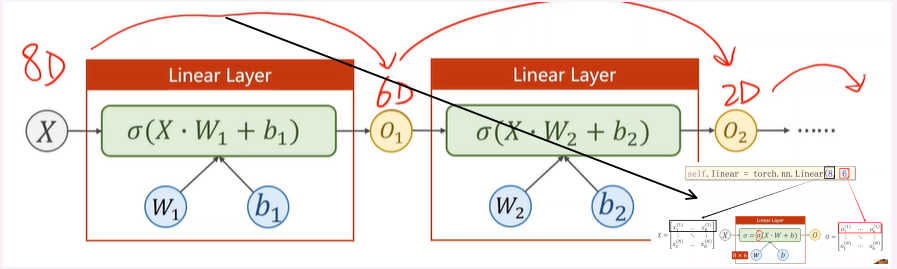对于程序编写来说，多维特征的数据输入，只在数据集构造和模型构造的代码上会有些许的改动。

#多维输入的模型构造
class Model(torch.nn.Module):
def __init__(self):
super(Model,self).__init__()
self.linear = torch.nn.Linear(8,1) #明确了输入数据是8维的，输出数据是1维的，从而定义了权重矩阵的维度
self.sigmoid = torch.nn.Sigmoid() #增加非线性映射

def forward(self,x):
y_pred = self.sigmoid(self.linear(x))
return y_pred

model = Model()


应用实例——构建网络度实现糖尿病病情预测

【需求描述】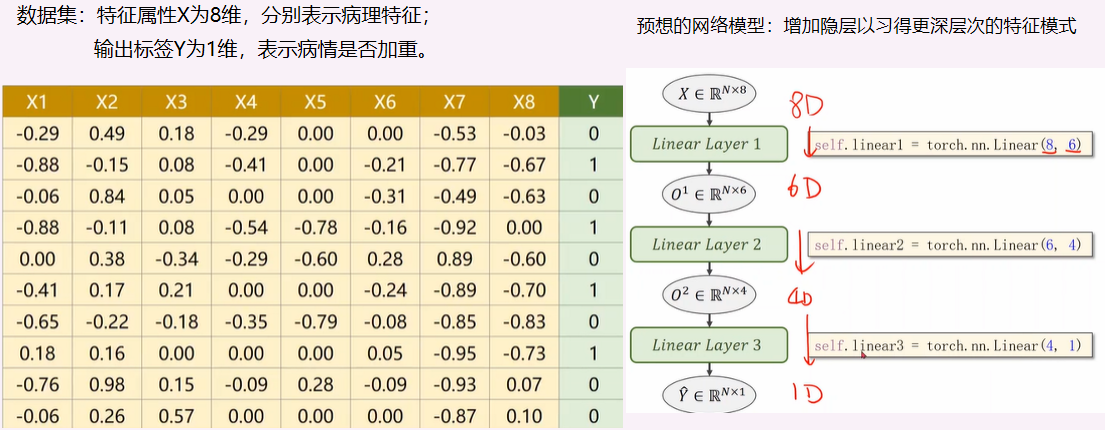【功能实现】
①在准备数据集上，注意把导入的数据集的X值和Y标签进行区分；

②在构建模型上，这里使用的Sigmoid是直接继承自nn.Module模块下的一个模块；作为一个非线性映射层，是可以在后面构建计算图的时候进行复用的；

③虽然数据维度和网络结构发生了变化，但因为最后的输出仍然是一维的概率值，所以损失函数和优化器和之前的单层逻辑回归相比，并未发生改变。

import numpy as np
import torch
import matplotlib.pyplot as plt

#准备数据

#自定义多层模型
class Model(torch.nn.Module):
def __init__(self):
super(Model,self).__init__()
self.linear1 = torch.nn.Linear(10,8)
self.linear2 = torch.nn.Linear(8,6)
self.linear3 = torch.nn.Linear(6,4)
self.linear4 = torch.nn.Linear(4,1)
self.sigmoid = torch.nn.Sigmoid() #增加非线性映射

def forward(self,x):
#在多隐层的网络中书写前向计算的逻辑时，只用一个变量x串联整个输入输出
#这是一种习惯
x = self.sigmoid(self.linear1(x))
x = self.sigmoid(self.linear2(x))
x = self.sigmoid(self.linear3(x))
x = self.sigmoid(self.linear4(x))
return x

model = Model()

#构建损失函数的计算和优化器
criterion = torch.nn.BCELoss(size_average = True)#逻辑回归模型适用二分类交叉熵损失函数
op = torch.optim.SGD(model.parameters(),lr = 0.1)

epochs = []
costs = []
#训练过程，对每个优化器都进行尝试
for epoch in range(1000):
epochs.append(epoch)
# 前向计算
y_pred = model(x_data)
loss = criterion(y_pred, y_data)
costs.append(loss.item())
print(epoch, loss.item())

# 反向传播
loss.backward()

#权重更新
op.step()

# 训练过程可视化
plt.ylabel('Cost')
plt.xlabel('Epoch')
plt.plot(epochs, costs)
plt.show()

数据集加载

在神经网络训练过程中采用的工具类，诸如Dataset 和 DataLoader

Dataset主要用于构造数据集，该数据集应该能够支持索引结构；
DataLoader主要用于加载数据集，支持训练时的Mini-Batch形式。

讲述上面的工具类，主要原因是在训练过程的Mini-Batch形式，该形式是介于Batch梯度下降和随机梯度下降之间的一种数据集加载方式，可以较好地兼顾到训练的随机性和时间的效率性。

在Mini-Batch模式下，训练过程要写成嵌套循环的形式

for epoch in range(training_epochs):
#对每一个mini-batch进行遍历
for i in range(total_batch):
# do something


外层循环控制迭代的次数
内层循环控制mini-bacth的索引

概念辨析

①Epoch

One forward pass and one backward pass of all the training examples
也即，所有的数据样本都参与了一次训练，就称为是一次Epoch

②Batch-size

The number of training examples in one forward backward pass
也即，每次进行一轮训练时所投入的训练样本的规模

③Iteration

Number of passes,each pass using [batch size]number of examples
通俗来说，就是上面嵌套循环中内层循环执行的次数，iteration=训练样本总数/batch-size

Dataset构造

①在torch.utils.data中有Dataset抽象类，我们需要根据自己的需要继承这个抽象类，来实现自己的数据集类；
②构造的数据集类中需要实现一些魔术方法：

init:对数据集类进行初始化
getitem:对数据集进行索引操作
len:对数据集中的数据项个数进行返回

③数据集的数据加载方式（init和getitem的实现）

若数据集本身规模不是很大，可以直接将原数据集全部加载到内存中，则getitem方法只需要从内存中顺序读出即可；
若数据集本身规模较大，则可以将数据在磁盘中按照文件夹分装，将输入出的各个文件夹名以列表的形式载入内存中；则getitem的方法就是按照文件夹路径从磁盘中索引并读出文件。

import torch
from torch.utils.data import Dataset

#准备数据,自定义数据集类，用以继承Dataset抽象类
class DiabetesDataset(Dataset):
def __init__(self):
pass
def __getitem__(self, item):
#该方法的实现是为了实例化后的数据集对象支持下标操作
#dataset[index]
pass
def __len__(self):
#该方法的实现是为了方便我们输出数据集每一个batch的数据项条目数
pass

dataset = DiabetesDataset()#实例化这个数据集类


②对原始数据集中的各个数据项进行随机打乱操作（Shuffle）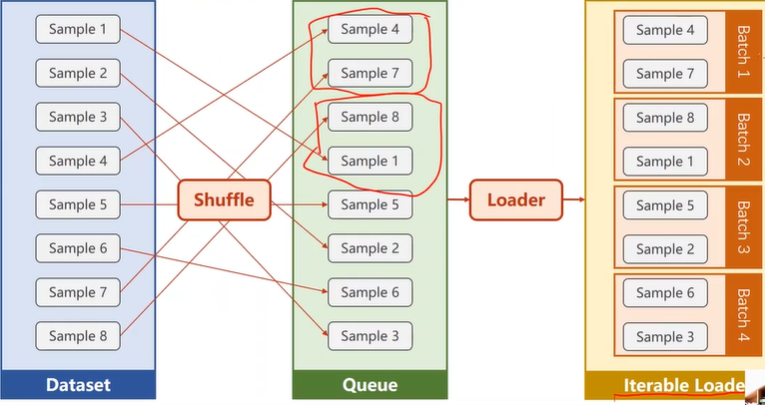train_loader = DataLoader(dataset=dataset,batch_size=32,shuffle=True,num_workers=2)
'''
dataset:数据集对象
batch_size:批量训练的大小
shuffle:是否要进行随机打乱
num_workers:使用多少个线程对数据进行载入操作；使用并行化可以提高数据读取的效率
'''


使用Dataset和DataLoader进行糖尿病分类

import numpy as np
import torch
import matplotlib.pyplot as plt

#准备数据,自定义数据集类，用以继承Dataset抽象类
class DiabetesDataset(Dataset):
def __init__(self):
#xy = np.loadtxt(filepath,delimiter=' ',dtype = np.float32)
self.len = self.y_data.shape
def __getitem__(self, index):
#该方法的实现是为了实例化后的数据集对象支持下标操作
return self.x_data[index],self.y_data[index]
def __len__(self):
#该方法的实现是为了方便我们输出数据集每一个batch的数据项条目数
return self.len

dataset = DiabetesDataset()#实例化这个数据集类
'''
dataset:数据集对象
batch_size:批量训练的大小
shuffle:是否要进行随机打乱
num_workers:使用多少个线程对数据进行载入操作；使用并行化可以提高数据读取的效率
'''

#自定义多层模型
class Model(torch.nn.Module):
def __init__(self):
super(Model,self).__init__()
self.linear1 = torch.nn.Linear(10,8)
self.linear2 = torch.nn.Linear(8,6)
self.linear3 = torch.nn.Linear(6,4)
self.linear4 = torch.nn.Linear(4,1)
self.sigmoid = torch.nn.Sigmoid() #增加非线性映射

def forward(self,x):
#在多隐层的网络中书写前向计算的逻辑时，只用一个变量x串联整个输入输出
#这是一种习惯
x = self.sigmoid(self.linear1(x))
x = self.sigmoid(self.linear2(x))
x = self.sigmoid(self.linear3(x))
x = self.sigmoid(self.linear4(x))
return x

model = Model()

#构建损失函数的计算和优化器
criterion = torch.nn.BCELoss(size_average = True)#逻辑回归模型适用二分类交叉熵损失函数
op = torch.optim.SGD(model.parameters(),lr = 0.01)

epochs = []
costs = []
#训练过程，包括前向计算和反向传播
for epoch in range(100):
epochs.append(epoch)
loss_sum = 0.0
inputs,labels = data
#从数据data中提取属性x和标签y
#并且自动转换成tensor张量传给inputs和data两个变量

y_pred = model(inputs)
loss = criterion(y_pred,labels)
loss_sum += loss.item()
print(epoch,i,loss.item())

loss.backward()

op.step()
costs.append(loss_sum/(i+1))

# 训练过程可视化
plt.ylabel('Cost')
plt.xlabel('Epoch')
plt.plot(epochs, costs)
plt.show()


p.s.P7和P8糖尿病分类的例子，我得到的损失函数值都是很大的负数，而且得到的loss-epoch曲线也很不正常，如果有遇到且解决了这个问题的小伙伴还请说明一下！！

多分类问题

多分类的输出处理

有m种可能的输出标签，就将最后一层设计成有m个输出，每个输出都表示该数据是其中某一类标签的可能性；
为了方便比较，输出的值应该满足某种分布的性质——每个输出值为正；输出值的总和为1；

也就是说，在分类问题中，必须满足输出的每一个值都代表着数据的分布；
p.s.在二分类问题中，经过sigmoid函数后输出一个值，其实就默认满足了分布的性质（p∈(0,1)）

Softmax层的计算

经过上面的分析，我们在多分类问题中得到的输出前加上一个softmax层，就可以满足上方关于输出值的限定。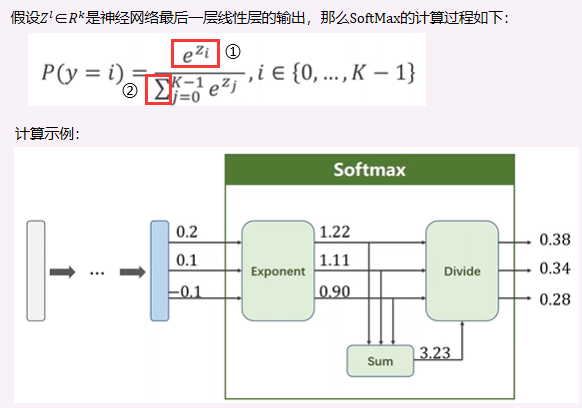①：将每一个输出值Zi都采用指数幂的运算，保证结果都是正数；
②：分母是每个指数幂结果的求和，保证最后输出的K个结果的总和为1（本质就是归一化操作）。

损失函数计算

主要思想还是参照二分类中的交叉熵损失函数；但要注意的是交叉熵的计算公式-(ylogy+(1-y)log(1-y))中虽然是有两项，但实际计算中有一项的值恒为零；
参照交叉熵的思想，我们对多分类的标签进行独热编码（one-hot），即目标标签值形如[0,0,…0,0,1,0,…,0]这样的稀疏向量；因此损失函数实际为-ylogy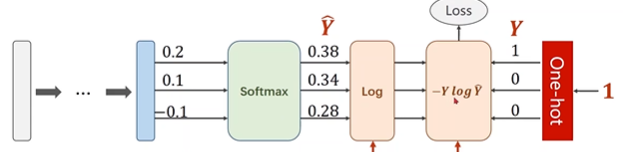#numpy实现多分类交叉熵损失函数
y = np.array([1,0,0]) #标签值，说明该数据是第1类
z = np.array([0.2,0.1,-0.1]) #神经网络最后一层线性单元的输出值
y_pred = np.exp(z) / np.exp(z).sum() #Softmax层的输出值
loss = (-y * np.log(y_pred)).sum()

#pytorch框架中对多分类损失函数的调用
y = torch.LongTensor()
z = torch.Tensor([0.2,0.1,-0.1])
criterion = torch.nn.CrossEntropyLoss()
loss = criterion(z,y)
#只需要声明一个损失函数计算对象
#传入标签值和线性单元输出值即可


Exercise9-1：CrossEntropyLoss v.s. NLLLoss
对两类损失函数的完整计算过程的理解可以参考这篇博客《Pytorch详解NLLLoss和CrossEntropyLoss》

①NLLLoss()
torch.nn.NLLLoss(weight=None, size_average=None, ignore_index=-100, reduce=None, reduction='mean')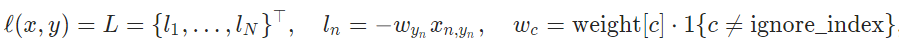也就是，损失向量l(x,y)取的是结果矩阵中标签id列所在的计算值；
②EntropyLoss()
torch.nn.CrossEntropyLoss(weight=None, size_average=None, ignore_index=-100, reduce=None, reduction='mean')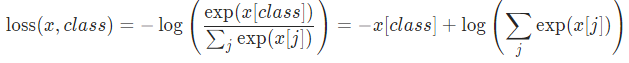直观理解交叉熵损失函数的计算过程，就是将得到的神经元输出值，统一进行指数幂的运算，再进行归一化得到概率分布结果，最后对归一化后的结果计算对数值。
利用对数运算的特性，看上图中第二个等式的表示，相当于对于每一个数据样本，log(Σexp(x[j]))都是需要计算的，唯一变化的就是-x[class]——也就是计算结果中和标签id对应的列标处的值。
即：CrossEntropy↔LogSoftMax+NLLLoss

案例实现——MNIST手写数字分类

import torch
from torchvision import transforms
from torchvision import  datasets
import torch.nn.functional as F
import matplotlib.pyplot as plt

#准备数据,转换成张量类型的数据，并进行归一化操作
batch_size = 64
transform = transforms.Compose([
transforms.ToTensor(),
transforms.Normalize((0.1307),(0.3081))
])
train_dataset = datasets.MNIST(root = "../dataset/mnist",

test_dataset = datasets.MNIST(root = "../dataset/mnist",train = False,

#自定义多层模型
class Net(torch.nn.Module):
def __init__(self):
super(Net,self).__init__()
self.linear1 = torch.nn.Linear(784,512)
self.linear2 = torch.nn.Linear(512,256)
self.linear3 = torch.nn.Linear(256,128)
self.linear4 = torch.nn.Linear(128,64)
self.linear5 = torch.nn.Linear(64,10)

def forward(self,x):#使用ReuLU激活单元
x = x.view(-1,784)
x = F.relu(self.linear1(x))
x = F.relu(self.linear2(x))
x = F.relu(self.linear3(x))
x = F.relu(self.linear4(x))
return self.linear5(x)#最后一层线性层的结果直接输出，不再激活

model = Net()

#构建损失函数的计算和优化器
criterion = torch.nn.CrossEntropyLoss()#多分类交叉熵损失函数
op = torch.optim.SGD(model.parameters(),lr = 0.01)#采用SGD

#训练过程，包括前向计算和反向传播，封装成一个函数
def train(epoch):
running_loss = 0.0
inputs,target = data

#前向计算
outputs = model(inputs)
loss = criterion(outputs,target)
#反向传播与权值更新
loss.backward()
op.step()

running_loss += loss.item()
if batch_idx % 300 == 299:#每训练300代就输出一次
print('[%d,%5d] loss: %3f' % (epoch+1,batch_idx+1,running_loss / 300))
running_loss = 0.0

#测试过程，封装成函数
def vali():
correct = 0
total = 0
images,labels = data
outputs = model(images)
_,predicted = torch.max(outputs.data,dim = 1)#因为是按批给的数据
#所以得到的数据标签也是一个矩阵
total += labels.size(0) #同样labels也是一个Nx1的张量
correct += (predicted == labels).sum().item()
print('Accuracy on test set: %d %%'%(100 * correct / total))

#主函数逻辑
if __name__ == '__main__':
for epoch in range(10): #一共训练10epochs
train(epoch)
vali()



课后作业——ottp电商产品多分类模型

实验过程中比较费力的几个点：

多分类交叉熵损失函数计算的本质用到了独热编码的思想，但是在实际调用过程中无需手动将标签值转换成独热编码；
train.csv文件的标签值是字符串类型，需要转换成数字类型
训练过程中的数据类型及其转换要格外注意

import numpy as np
import torch
import torch.nn.functional as F
import pandas as pd

#定义数据集
class OttoProduct(Dataset):
def __init__(self):
x = np.loadtxt('../dataset/otto/train.csv',delimiter=',',skiprows = 1,usecols = list(range(1,94)))
self.len = x.shape
self.x_data = torch.from_numpy(x)
y = pd.get_dummies((np.loadtxt('../dataset/otto/train.csv', delimiter=',', dtype = str, skiprows=1,
usecols=94)))#转换成独热编码
self.y_data = torch.from_numpy(np.argmax(y.values,axis = 1))

def __getitem__(self, index):
return self.x_data[index],self.y_data[index]

def __len__(self):
return self.len
dataset = OttoProduct() #实例化数据集
# print(dataset.x_data[:10])
print(dataset.y_data[:10])

#定义分类网络结构
class Net(torch.nn.Module):
def __init__(self):
super(Net,self).__init__()
self.l1 = torch.nn.Linear(93,64)
self.l2 = torch.nn.Linear(64,32)
self.l3 = torch.nn.Linear(32,9)

def forward(self,x):
x = x.view(-1,93)
x = F.relu(self.l1(x))
x = F.relu(self.l2(x))
return self.l3(x)
model = Net()#实例化网络

#构建损失函数和优化器
criterion = torch.nn.CrossEntropyLoss()
op = torch.optim.SGD(model.parameters(),lr = 0.01,momentum = 0.5)

#训练过程
def train(epoch):
running_loss = 0.0
inputs,target = data

#前向计算
inputs = torch.tensor(inputs,dtype = torch.float32)
outputs = model(inputs)
# print(outputs)
loss = criterion(outputs,target)

#反向传播与权值优化
loss.backward()
op.step()

running_loss += loss.item()
if batch_idx % 500 == 499:#每训练500iterations就打印出结果
print('[%d,%5d] loss: %3f'%(epoch+1,batch_idx+1,running_loss / 500))
running_loss = 0.0

#主函数逻辑
if __name__ == '__main__':
for epoch in range(10):#一共训练10个epochs
train(epoch)




展开全文逻辑回归 DataLoader
• pytorch深度学习PyTorch has sort of became one of the de facto standards for creating Neural Networks now, and I love its interface. Yet, it is somehow a little difficult for beginners to get a hold of...机器学习 人工智能 python java
• #设置输入维数、输出维数、训练次数、学习率 input_size = 1 output_size = 1 num_epochs = 60 learning_rate = 0.001 #定义训练集数据 x_train = np.array([[3.3], [4.4], [5.5], [6.71], [6.93], [4.168机器学习 神经网络
• Ubuntu 20.04安装及pytorch深度学习框架配置Ubuntu 20.04安装启动U盘制作安装ubuntu 20.04功能快捷键合理的创建标题，有助于目录的生成如何改变文本的样式插入链接与图片如何插入一段漂亮的代码片生成一个适合你的...linux python ubuntu
• 基于Pytorch深度学习的新冠肺炎的CT图像识别python 机器学习 人工智能 java
• 其中，PyTorch作为后起之秀，发展非常迅猛，在学术界逐渐占据垄断地位，许多计算机顶级期刊、会议的最新文章的源代码都采用PyTorch深度学习框架。 因此，为了帮助广大科研人员更加系统地学习人工智能（包括传统机器...python 算法
• PyTorch安装指令 请先安装Anaconda和CUDA 10.0。 配置国内源 # 配置国内源，方便安装Numpy,Matplotlib等 conda config - - add channels ...
• 文章目录基于PyTorch的两层神经网络一、基于numpy的两层神经网络实现：二、基于PyTorch的两层神经网络实现：三、...本案例中实现的两层神经网络如下图所示：其中输入X为1000维的向量，输出Y为10维的向量。而中间隐藏层
• 很久没有碰Pytorch了，准备以实战项目代码回顾的方式进行复习。 2.Pytorch安装 现在我又切回了ubuntu系统，里面没有Pytorch，所以顺便从Pytorch最新版安装开始讲起吧。 （1）本机配置 CUDA9.0, CUDNN7.1.2 Anaconda...
• 目录1. earlystop1.1简介1.2 如何使用早停法1.2.1、停止标准简介1.2.2、停止标准...但是所有的标准深度学习神经网络结构如全连接多层感知机都很容易过拟合：当网络在训练集上表现越来越好，错误率越来越低的时候，实python 机器学习
• 2、模型选择 模型我们已经选择完了，就用上篇文章《Pytorch深度学习实战教程（二）：UNet语义分割网络》讲解的 UNet 网络结构。 但是我们需要对网络进行微调，完全按照论文的结构，模型输出的尺寸会稍微小于图片...语义分割 人工智能
• 本文你将会了解到1、如何使用PyTorch对数据集进行导入 2、如何使用PyTorch搭建一个简易的深度学习模型 3、如何优化和训练我们搭建好的模型 注：本案例使用的Py...
• 深度学习为智能系统提供了动力，随着GPU和PyTorch、Keras、TensorFlow的进步以及大数据的可用性，在文本、视觉和分析等领域更容易实施相应问题的解决方案。 《深度学习原理与实践》电子资料详细介绍了目前深度学习...python
• plt.show() 运行结果 写在最后 本文章为《PyTorch深度学习实践》完结合集课程对应的一些课后习题解答，仅为各位同志学习参考之用 各位看官，都看到这里了，麻烦动动手指头给博主来个点赞8，您的支持作者最大的创作...机器学习 人工智能 神经网络
• 又到了一周送书的时间啦！本周的送书《TensorFlow+PyTorch深度学习从算法到实战》（赠书规则见文末）作者介绍作者简介：刘子瑛，阿里巴巴操作系统框架专家；CSDN 博客专家。工...
• 在第一次学习过程中，先利用Numpy实现一个简单的机器学习案例。 我们已经知道，机器学习需要我们去训练出一个模型。而在我们中学求导时，总会有这么一道题，给定几个点，让我们去通过这几个点求一个函数的方程式。...python
• 一、前言该系列文章的内容有：Pytorch的基本使用语义分割算法讲解先从最简单的语义分割基础与开发环境搭建开始讲解。PS：由于微信不允许外部链接，你需要点击页尾左下角的“阅读原文”，才能...
• Pytorch深度学习（2） -- RNN及其进阶模型实现0 预测训练函数1.RNN实现RNN小结2.GRU实现GRU小结3.LSTM实现LSTM小结4.Deep-RNN加入参数num_layers=25.Bi-Rnn实现加入参数bidirectional=True 0 预测训练函数 def train...
• 本课程从卷积神经网络CNN 开始讲起，逐步延伸到深度学习各大神经网络，全程原理和案例代码实战，一步步带大家入门如何使用Pytorch玩转深度学习。 课程风格通俗易懂，快速掌握当下最热门的深度学习框架！最后通过 ...人工智能 框架
• 手写数字识别分类案例代码： 引入的包和库： 将图像转换为pytorch的tensor 将图像归一化为0-1分布 将transforms运用到dataset中： 构建模型： 定义损失函数和优化器： 训练： 卷积神经网...
• 在我们学习深度学习的过程中，往往需要大数据，深网络。但有时我们很难同时获取这些条件。这时我们可以使用其他任务开发的模型来应用于我们自己的任务。而这，就是迁移学习。 迁移学习是机器学习的一种方法，可以将......